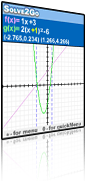Solve2Go 0.84Solve2Go supports solving equations and inequalities conjectures based on visual thinking. Conjectures can be refuted or supported by examples provided by the tool, and should be proved using symbolic manipulations on paper.

### Description

Solve2Go supports solving equations and inequalities by means of conjectures based on visual thinking. Conjectures can be refuted or supported by examples provided by the tool, and should be proved using symbolic manipulations on paper.

In many mathematical investigations we encounter the need to compare two functions. Solve2Go supports comparisons of two types:

Equations: when we want to know for which values of x the two functions are equal.

Inequalities: when we want to know for which values of x one function is greater than the other.

When the two functions involved are linear, we call the comparison a linear comparison. When at least one of the functions is not linear, we refer to a non-linear comparison. Non-linear comparisons form a wide and rich field of study

### Features

Users specify two function expressions by choosing each expression from a list of given parametric function expressions. Solve2Go randomly chooses numeric values for the parameters and graphs the two functions. It also marks points of intersection when they exist and are visible on screen.

To explore solutions of other equations or inequalities of the same (selected) type, it is recommended to use the interactive change of the constants and coefficients in each expression, transform the graphs, and view whether and how solutions are changing. The design of Solve2Go is based on the special features of Graph2Go (see the Graph2Go features).

### Suggested Activities

Create a Gallery

Use Solve2Go to examine different common positions between a quadratic function and a linear function, and between two quadratic functions. How would you present a “gallery” of different comparisons to give a “visitor” a general picture of various possible types of comparisons?

Consider the following properties of comparisons:

• The type of functions compared (quadratic, linear, constant, increasing…).
• The type of comparison (equation, inequality…).
• The nature of the set of solutions (no solutions, a single solution, several solutions, an interval of solutions, several intervals of solutions).

Investigation problems

Problems in context

Library of exercises